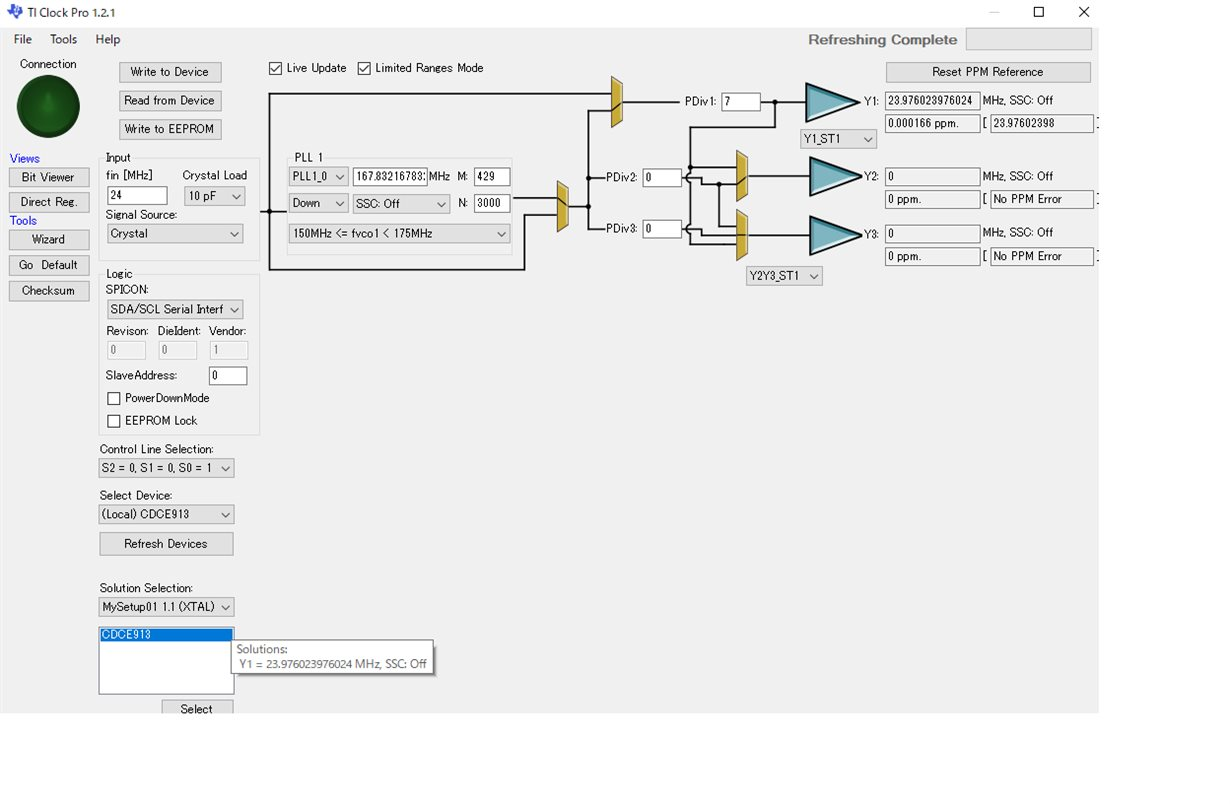If you have a related question, please click the "Ask a related question" button in the top right corner. The newly created question will be automatically linked to this question.

# CDCE913: M value calculation from ClockPro

Part Number: CDCE913
Other Parts Discussed in Thread: CLOCKPRO

Hello

Q1

If I set fin = 24MHz , and if I choose fout= 24* 1000/1001 =23.97602397MHz,

I can calculate from ClockPro as  fout = 24 * 3000 /3003 = 24* 3000/( 7* 429 ) ;  Pdiv=7, N=3000, M=429.

Therefore, fout should be 23.97602397MHz ( 0ppm) but Clock Pro putput is 23.976023976024 ( 0.000166ppm error).

Why does it occur ?

I guess M value using in ClockPro is something wrong..

Q2

How does Clock Pro calculate M value from N,P,Q,R?

We see N,P,Q,R value ( 18~1Bh, 1C~1Fh), but M value is not defined in the register setting.

How does Clock Pro get M value?

I worried about even I set N,P,Q,R correctly , I might have some error% in fout value.Best Regards

• Hello Shibatani,

Q1.

Your set frequency is 23.97602398MHz = 23,987,023.98Hz

ClockPro calculation frequency is 23.976023976024 MHz = 23,976,023.97024Hz

The idea is that the set frequency you had set is a repeating number and you rounded off the number at  nearest hundreth of Hz, calculate again and you will receive the same frequency as ClockPro but there is a slight error in ppm due to your rounding and ClockPro's exact calculated number.

The ppm error is 0.0000166ppm = 0.0166ppb = 16.6ppt. At this inaccuracy this could be part to part variation of crystals and this setting works fine for your application.

Q2.

Here are the calculation as well as bit definitions of:

N = PLL1_xN[11:0] 12 bit register 0<N<4096

P = 4 – int(log2N / M)  = PLL1_0P[2:0] 3 bit register 0<=P<=7

Q = int(N' / M) = PLL1_xQ[5:0] 6 bit register 16<= Q<=63

R = N′ – M × Q = PLL1_xR[8:0] 9 bit register 0<=R<=511

These registers define values for P, Q, and R which have parameters that must be met, therefore determining if there is a good sequence for N/M ratio. This is why when trying to change M or N values you might cause unintentional F_vco ranges outside of parameters. Please use ClockPro Wizard for Setup generation if you are trying to calculate for a frequency combination.

• Dear Sirs,

In regard to this post. please forget.

I had mistake that the value was rounded.

24* 1000/1001 = 23.976023976024 should be  correct.

I 'm sorry.